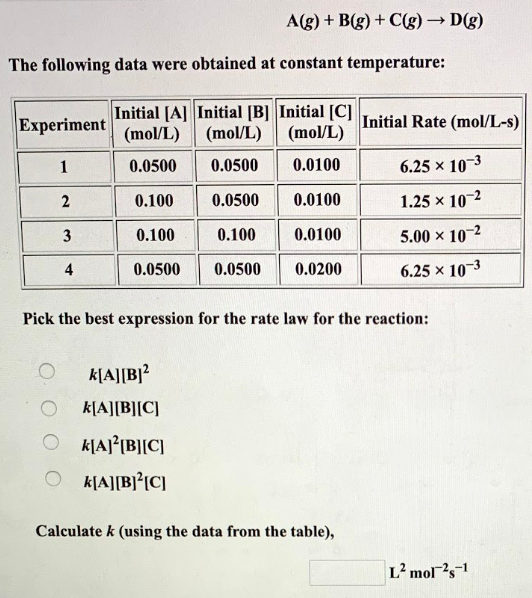# A(g) + B(g) + C(g) → D(g) The following date were obtained at a constant temperature: Pick the best answer expression for the rate law for the reaction: a) k[A][B]^2 b) k[A][B][C] c) k[A]^2[B][C] d) k[A][B]^2[C] Calculate k (using the data from the table),